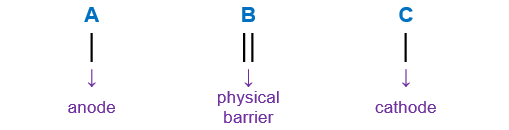# Problem: A 1M solution of Cu ( NO3 )2 is placed in a beaker with a strip of Cu metal. A 1 M solution of SnSO4 is placed in a second beaker with a strip of Sn metal. A salt bridge connects the two beakers, and wires to a voltmeter link the two metal electrodes.Write the equation for the overall cell reaction.

###### FREE Expert Solution

We’re being asked to write the overall balanced equation for a Cu and Sn galvanic cell:

The half cell reactions for Cu and Sn (these potentials can be searched from the internet):

Cu 2+(aq) + 2e- → Cu(s) E = 0.337 V
Sn 2+ (aq) + 2e- → Sn(s) E = -0.136 V

The Anode is where oxidation takes place while the cathode is where reduction takes place. The larger (more +) the reduction potential value is, the more likely that would correspond to reduction and vise versa for oxidation.

Sn will be the anode and Cu will be the cathode reaction.

So the cell notation for the reaction is:

Sn(s) | Sn2+(aq, 1.0 M) || Cu2+(aq, 1.0 M)| Cu(s)

When writing a cell notation, we use the following format – “as easy as ABCRecall the mnemonics LEO GER.

Lose               Gain
Electron         Electrons
Oxidation       Reduction

lose electrons  oxidation → anode
gain electrons  reduction → cathode

We’re going to write the overall balanced reaction equation using the following steps:

Step 1: Write the anode reaction.
Step 2: Write the cathode reaction.
Step 3: Balanced anode and cathode reactions.
Step 4: Get the overall reaction.

93% (406 ratings)###### Problem Details

A 1M solution of Cu ( NO3 )2 is placed in a beaker with a strip of Cu metal. A 1 M solution of SnSO4 is placed in a second beaker with a strip of Sn metal. A salt bridge connects the two beakers, and wires to a voltmeter link the two metal electrodes.

Write the equation for the overall cell reaction.